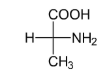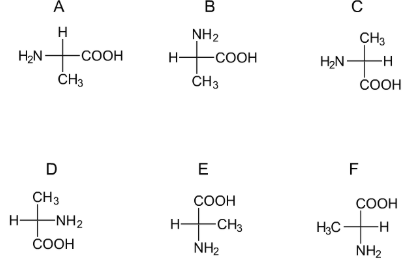# Problem: Alanine is shown below as a Fischer projection. Identify the stereochemistry configuration.a) Rb) SWhich Fischer structures below have the same stereochemistry configuration?a) Ab) Bc) Cd) De) Ef) F

###### FREE Expert Solution

We are asked to identify the stereochemistry configuration of the given Ficher Projection.We can see that this is a chiral molecule because:

– there is no plane of symmetry present

– there is a chiral center present

Recall that the R/S configuration gives us the absolute stereochemistry of chiral centers. It involves:

Step 1:  Determine the priority of groups at the chiral center.

• Priority is determined using atomic mass.

• If the same atom is present, we compare the other atoms it is bonded to.

– Double and triple bonds count twice/thrice.

Step 2: Determine the R/S configuration of the chiral center.

• If the lowest priority is on a dashed line: trace a path from 1 to 3

– If the order is clockwise R (rectus or right)

– If the order is counterclockwise S (sinister or left)###### Problem Details

Alanine is shown below as a Fischer projection. Identify the stereochemistry configuration.a) R

b) S

Which Fischer structures below have the same stereochemistry configuration?a) A

b) B

c) C

d) D

e) E

f) F